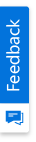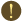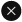We use cookies to give you the best experience on our website. If you continue to browse, then you agree to our privacy policy and cookie policy.Unfortunately, activation email could not send to your email. Please try again.# Format Excel chart data label in C#, VB.NET

Platform: WinForms |
Control: XlsIO

Syncfusion Excel (XlsIO) library is a .NET Excel library used to create, read, and edit Excel documents. Also, converts Excel documents to PDF files. Using this library, you can format Excel chart data label.

Data labels make a chart easier to understand because they show details about a data series or its individual data points. There are a lot of formatting options for data labels. You can format font style, size, border style and apply specific colors.

## Steps to format Excel chart data label, programmatically:

Step 1: Create a new C# console application project.Create a new C# console application

Step 2: Install the Syncfusion.XlsIO.WinForms NuGet package as reference to your .NET Framework application from NuGet.org.Install NuGet package

Step 3: Include the following namespace in the Program.cs file.

C#

```using Syncfusion.XlsIO;
```

VB.NET

```Imports Syncfusion.XlsIO
```

Step 4: Use the following code snippet to format Excel chart data label in C#, VB.NET.

C#

```using (ExcelEngine excelEngine = new ExcelEngine())
{
//Instantiate the application object
IApplication application = excelEngine.Excel;

IWorkbook workbook = application.Workbooks.Create(1);

//The first worksheet object in the worksheets collection is accessed
IWorksheet worksheet = workbook.Worksheets;
worksheet.Name = "First Chart";

//Entering the chart data
worksheet.Range["A1"].Text = "Texas books Unit sales";
worksheet.Range["A1:D1"].Merge();
worksheet.Range["A1"].CellStyle.Font.Bold = true;

worksheet.Range["B2"].Text = "Jan";
worksheet.Range["C2"].Text = "Feb";
worksheet.Range["D2"].Text = "Mar";

worksheet.Range["A3"].Text = "Austin";
worksheet.Range["A4"].Text = "Dallas";
worksheet.Range["A5"].Text = "Houston";
worksheet.Range["A6"].Text = "San Antonio";

worksheet.Range["B3"].Number = 53.75;
worksheet.Range["B4"].Number = 52.85;
worksheet.Range["B5"].Number = 59.77;
worksheet.Range["B6"].Number = 96.15;

worksheet.Range["C3"].Number = 79.79;
worksheet.Range["C4"].Number = 59.22;
worksheet.Range["C5"].Number = 10.09;
worksheet.Range["C6"].Number = 73.02;

worksheet.Range["D3"].Number = 26.72;
worksheet.Range["D4"].Number = 33.71;
worksheet.Range["D5"].Number = 45.81;
worksheet.Range["D6"].Number = 12.17;

//Adding a New (Embedded chart) to the worksheet
IChartShape shape = worksheet.Charts.Add();
shape.ChartType = ExcelChartType.Column_Clustered;
shape.DataRange = worksheet.Range["B3:D6"];
shape.IsSeriesInRows = false;
shape.PrimaryCategoryAxis.Title = "City";
shape.PrimaryCategoryAxis.CategoryLabels = worksheet["A3:A6"];
shape.PrimaryValueAxis.Title = "Sales (in Dollars)";
shape.ChartTitle = "Texas Books Unit Sales";
shape.HasDataTable = true;

// Displaying the values in the series
IChartDataPoint point = shape.Series.DataPoints.DefaultDataPoint;
IChartDataLabels labels = point.DataLabels;

// Formatting the labels
labels.IsValue = true;
labels.Bold = true;
labels.FrameFormat.Border.LinePattern = ExcelChartLinePattern.DashDotDot;
labels.Size = 20;
labels.Italic = true;
labels.Color = ExcelKnownColors.Red;

//Save the file
workbook.SaveAs("Output.xlsx");

System.Diagnostics.Process.Start("Output.xlsx");
}
```

VB.NET

```Using excelEngine As ExcelEngine = New ExcelEngine()
'Instantiate the application object
Dim application As IApplication = excelEngine.Excel

Dim workbook As IWorkbook = application.Workbooks.Create(1)

'The first worksheet object in the worksheets collection is accessed
Dim worksheet As IWorksheet = workbook.Worksheets(0)
worksheet.Name = "First Chart"

'Entering the chart data
worksheet.Range("A1").Text = "Texas books Unit sales"
worksheet.Range("A1:D1").Merge()
worksheet.Range("A1").CellStyle.Font.Bold = True

worksheet.Range("B2").Text = "Jan"
worksheet.Range("C2").Text = "Feb"
worksheet.Range("D2").Text = "Mar"

worksheet.Range("A3").Text = "Austin"
worksheet.Range("A4").Text = "Dallas"
worksheet.Range("A5").Text = "Houston"
worksheet.Range("A6").Text = "San Antonio"

worksheet.Range("B3").Number = 53.75
worksheet.Range("B4").Number = 52.85
worksheet.Range("B5").Number = 59.77
worksheet.Range("B6").Number = 96.15

worksheet.Range("C3").Number = 79.79
worksheet.Range("C4").Number = 59.22
worksheet.Range("C5").Number = 10.09
worksheet.Range("C6").Number = 73.02

worksheet.Range("D3").Number = 26.72
worksheet.Range("D4").Number = 33.71
worksheet.Range("D5").Number = 45.81
worksheet.Range("D6").Number = 12.17

'Adding a New (Embedded chart) to the Worksheet
Dim shape As IChartShape = worksheet.Charts.Add()
shape.ChartType = ExcelChartType.Column_Clustered
shape.DataRange = worksheet.Range("B3:D6")
shape.IsSeriesInRows = False
shape.PrimaryCategoryAxis.Title = "City"
shape.PrimaryCategoryAxis.CategoryLabels = worksheet("A3:A6")
shape.PrimaryValueAxis.Title = "Sales (in Dollars)"
shape.ChartTitle = "Texas Books Unit Sales"
shape.HasDataTable = True

'Displaying the values in the series
Dim point As IChartDataPoint = shape.Series(0).DataPoints.DefaultDataPoint
Dim labels As IChartDataLabels = point.DataLabels

'Formatting the labels
labels.IsValue = True
labels.Bold = True
labels.FrameFormat.Border.LinePattern = ExcelChartLinePattern.DashDotDot
labels.Size = 20
labels.Italic = True
labels.Color = ExcelKnownColors.Red

'Save the file
workbook.SaveAs("Output.xlsx")

System.Diagnostics.Process.Start("Output.xlsx")
End Using
```

A complete Windows Forms working example of how to format Excel chart data label in C# and VB can be downloaded from Format Excel Chart Data Label.zip.

Refer here to explore the rich set of Syncfusion Excel (XlsIO) library features.

How to set multilevel labels for Excel chart axis in C#, VB.NET?

How to position and re-size a plot area of chart using XlsIO?

How to change the color of single Data point in XlsIO chart?

How to set position for multiple charts in a single sheet and how to display label names?

How to set the DataLabel position for chart series using XlsIO?

Note:

Starting with v16.2.0.x, if you reference Syncfusion assemblies from trial setup or from the NuGet feed, include a license key in your projects. Refer the link to learn about generating and registering Syncfusion license key in your application to use the components without trail message.

2X faster development

The ultimate WinForms UI toolkit to boost your development speed.You are using an outdated version of Internet Explorer that may not display all features of this and other websites. Upgrade to Internet Explorer 8 or newer for a better experience.